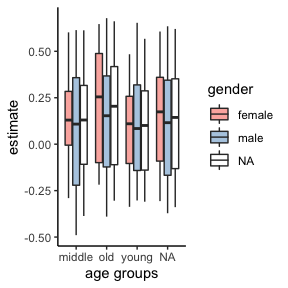# Visualizing specification curve analyses

This vignette exemplifies different ways to plot results from specification curve analyses. Since version 0.3.0, running the function specr() creates an object of class specr.object, which can be investigated and plotted using generic function (e.g., summary() and plot(). For most cases, simply wrapping the function plot() around the results will already create a comprehensive visualization. However, more specific customization is possible if we use specify the argument type (e.g., type = "curve"). Different types are available. All of resulting plots are objects of the class ggplot, which means they can be customized and adjusted further using the grammar of graphics provided by the package ggplot2. specr also exports the function plot_grid() from the package cowplot, which can be used to combine different types of plots.

# Specification curve analysis

## Setting up specifications

In order to have some data to work with, we will use the example data included in the package.

# Load packages
library(tidyverse)
library(specr)

# Setup specifications
specs <- setup(data = example_data,
y = c("y1", "y2"),
x = c("x1", "x2"),
model = "lm",
controls = c("c1", "c2"),
subsets = list(group1 = c("young", "middle", "old"),
group2 = c("female", "male")))

# Summary of the specification setup
summary(specs)
## Setup for the Specification Curve Analysis
## -------------------------------------------
## Class:                      specr.setup -- version: 1.0.0
## Number of specifications:   192
##
## Specifications:
##
##   Independent variable:     x1, x2
##   Dependent variable:       y1, y2
##   Models:                   lm
##   Covariates:               no covariates, c1, c2, c1 + c2
##   Subsets analyses:         young & female, middle & female, old & female, female, young & male, middle & male, old & male, male, young, middle, old, all
##
## Function used to extract parameters:
##
##   function (x)
## broom::tidy(x, conf.int = TRUE)
## <environment: 0x7fe4dacebb88>
##
##
## Head of specifications table (first 6 rows):
## # A tibble: 6 × 8
##   x     y     model controls      subsets         group1 group2 formula
##   <chr> <chr> <chr> <chr>         <chr>           <fct>  <fct>  <glue>
## 1 x1    y1    lm    no covariates young & female  young  female y1 ~ x1 + 1
## 2 x1    y1    lm    no covariates middle & female middle female y1 ~ x1 + 1
## 3 x1    y1    lm    no covariates old & female    old    female y1 ~ x1 + 1
## 4 x1    y1    lm    no covariates female          <NA>   female y1 ~ x1 + 1
## 5 x1    y1    lm    no covariates young & male    young  male   y1 ~ x1 + 1
## 6 x1    y1    lm    no covariates middle & male   middle male   y1 ~ x1 + 1

We can see that we will estimate 192 models.

## Fitting the models

Next, we fit the models across these 192 specifications. As this example won’t take much time, we do not parallelize and stick to one core (by setting workers = 1).

# Running specification curve analysis
results <- specr(specs)

## Investigating the results

Let’s quickly get some ideas about the specification curve by using the generic summary() function.

# Overall summary
summary(results)
## Results of the specification curve analysis
## -------------------
## Technical details:
##
##   Class:                          specr.object -- version: 1.0.0
##   Cores used:                     1
##   Duration of fitting process:    2.822 sec elapsed
##   Number of specifications:       192
##
## Descriptive summary of the specification curve:
##
##  median  mad   min  max   q25  q75
##    0.13 0.45 -0.49 0.68 -0.16 0.44
##
## Descriptive summary of sample sizes:
##
##  median min  max
##     250 160 1000
##
## Head of the specification results (first 6 rows):
##
## # A tibble: 6 × 26
##   x     y     model controls    subsets group1 group2 formula estimate std.error
##   <chr> <chr> <chr> <chr>       <chr>   <fct>  <fct>  <glue>     <dbl>     <dbl>
## 1 x1    y1    lm    no covaria… young … young  female y1 ~ x…     0.48      0.08
## 2 x1    y1    lm    no covaria… middle… middle female y1 ~ x…     0.6       0.1
## 3 x1    y1    lm    no covaria… old & … old    female y1 ~ x…     0.65      0.08
## 4 x1    y1    lm    no covaria… female  <NA>   female y1 ~ x…     0.61      0.05
## 5 x1    y1    lm    no covaria… young … young  male   y1 ~ x…     0.65      0.08
## 6 x1    y1    lm    no covaria… middle… middle male   y1 ~ x…     0.61      0.09
## # … with 16 more variables: statistic <dbl>, p.value <dbl>, conf.low <dbl>,
## #   conf.high <dbl>, fit_r.squared <dbl>, fit_adj.r.squared <dbl>,
## #   fit_sigma <dbl>, fit_statistic <dbl>, fit_p.value <dbl>, fit_df <dbl>,
## #   fit_logLik <dbl>, fit_AIC <dbl>, fit_BIC <dbl>, fit_deviance <dbl>,
## #   fit_df.residual <dbl>, fit_nobs <dbl>

The median effect size is b = 0.13 (mad = 0.45). Sample sizes range from 250 to 1000 (the full data set). We can also summarize different aspects in more detail. For example, by add type = "curve", we are able to produce descriptive summaries of the multiverse of parameters.

# Specific descriptive analysis of the curve, grouped by x and y
summary(results,
type = "curve",
group = c("x", "y"))
## # A tibble: 4 × 9
## # Groups:   x 
##   x     y       median    mad    min    max     q25     q75   obs
##   <chr> <chr>    <dbl>  <dbl>  <dbl>  <dbl>   <dbl>   <dbl> <dbl>
## 1 x1    y1     0.605   0.0446  0.446  0.678  0.589   0.638    250
## 2 x1    y2    -0.272   0.0905 -0.490 -0.124 -0.314  -0.207    250
## 3 x2    y1     0.229   0.0651  0.107  0.438  0.193   0.276    250
## 4 x2    y2    -0.00536 0.115  -0.204  0.184 -0.0784  0.0731   250

We see that it makes quite a difference what variables are actually used to estimate the relationship.

# Visualizations

The primary goal of this vignette is to provide some examples of how these results - and specifically the specification curve - can be visualized. Because received an object of class specr.object, we can create most visualizations using the generic plot() function. Different plots can be created by changing the argument type (e.g., type = "boxplot").

## Comprehensive standard visualization

The simplest way to visualize most of the information contained in the results data frame is by using the plot() function without any further arguments specified. In the background, the function is actually set to type = "default", which creates both the specification curve plot and the choice panel and combines them into a larger figure.

plot(results)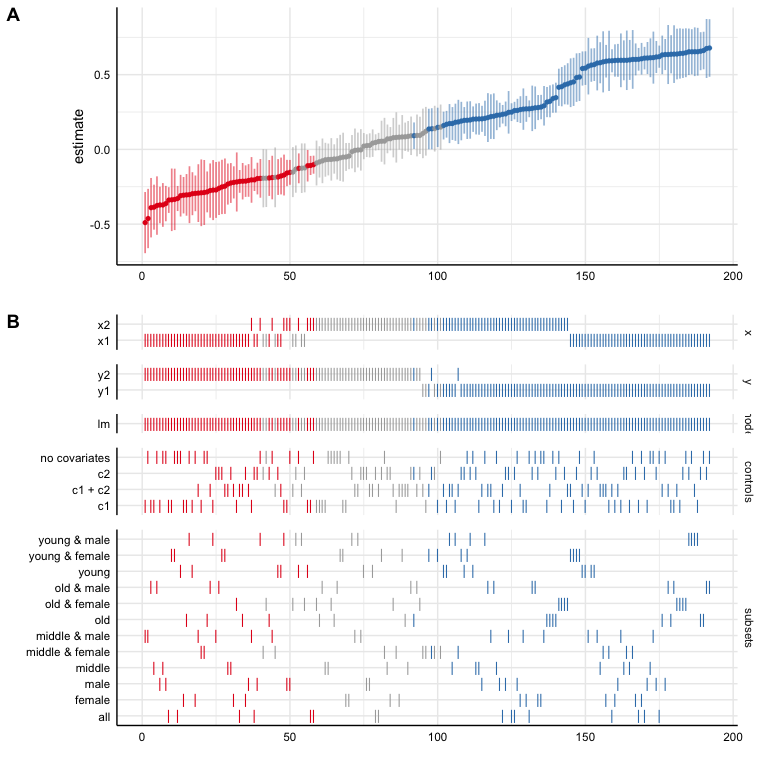We can further customize that function, e.g., by removing unnecessary information (in this case we only specified one model, plotting this analytical choice is hence useless) or by reordering/transforming the analytical choices (and thereby visualize specific contrasts).

# Customizing plot
plot(results,
choices = c("x", "y", "controls", # model is not plotted
"group1", "group2"),  # subset split into original groups
rel_heights = c(.75, 2))          # changing relative heights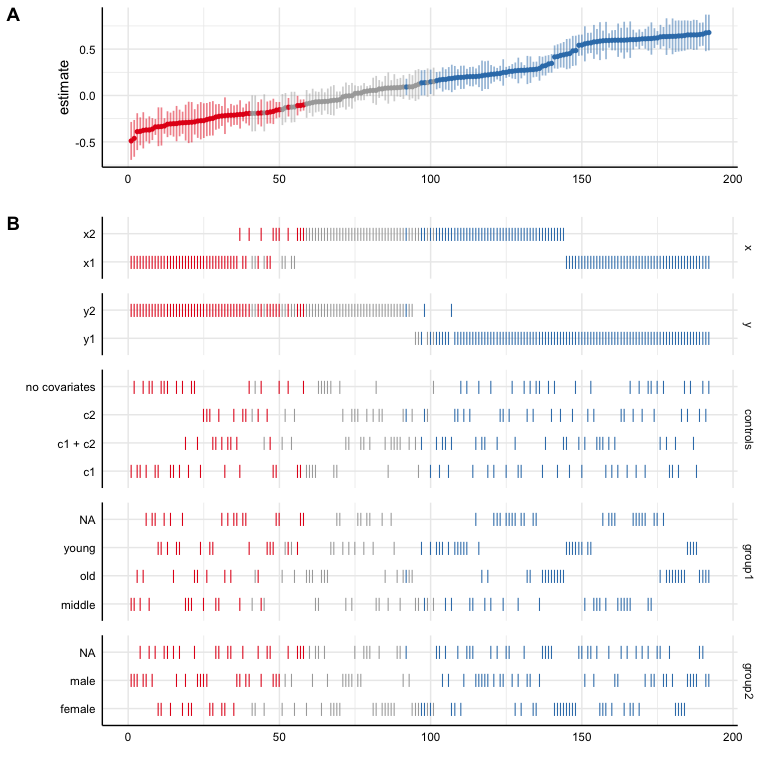# Investigating specific contrasts
results$data <- results$data %>%
mutate(gender = ifelse(grepl("female", subsets), "female",
ifelse(grepl("male", subsets), "male", "all")))

# New variable as "choice"
plot(results,
choices = c("x", "y", "gender"))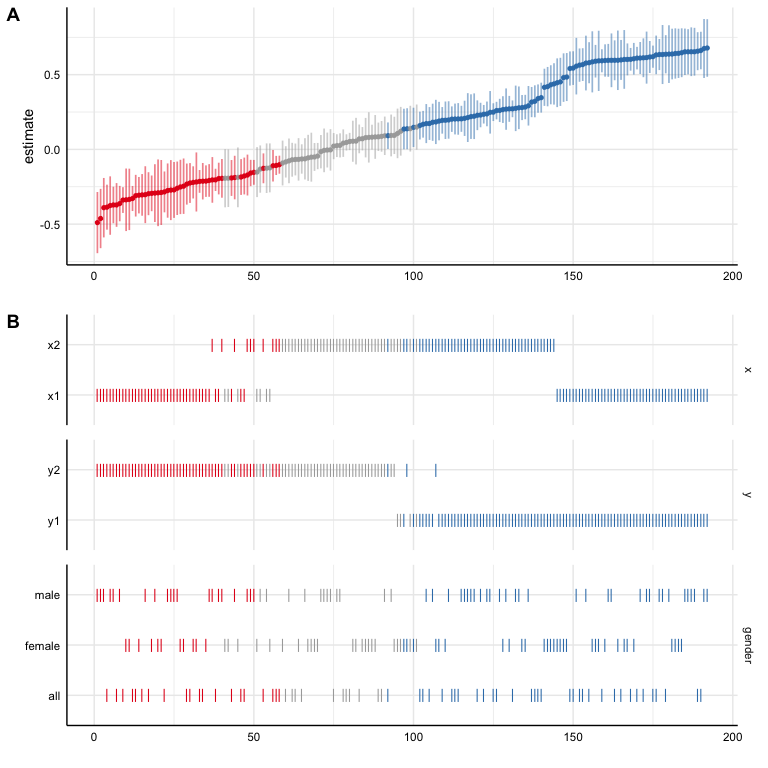### Plot curve and choices seperately

Using plot(x, type = "default") is not very flexible. It is designed to provided a quick, but meaningful visualization that covers the most important aspects of the analysis. Alternatively, we can plot the specification curve and the choice panel individually and bind them together afterwards. This is useful as it allows us to customize and change both individual plots (using ggplot-functions!)

# Plot specification curve
p1 <- plot(results,
type = "curve",
ci = FALSE,
ribbon = TRUE) +
geom_hline(yintercept = 0,
linetype = "dashed",
color = "black") +
labs(x = "", y = "unstd. coefficients")

# Plot choices
p2 <- plot(results,
type = "choices",
choices = c("x", "y", "controls")) +
labs(x = "specifications (ranked)")

# Combine plots (see ?plot_grid for possible adjustments)
plot_grid(p1, p2,
ncol = 1,
align = "v",             # to align vertically
axis = "rbl",            # align axes
rel_heights = c(2, 2))   # adjust relative heights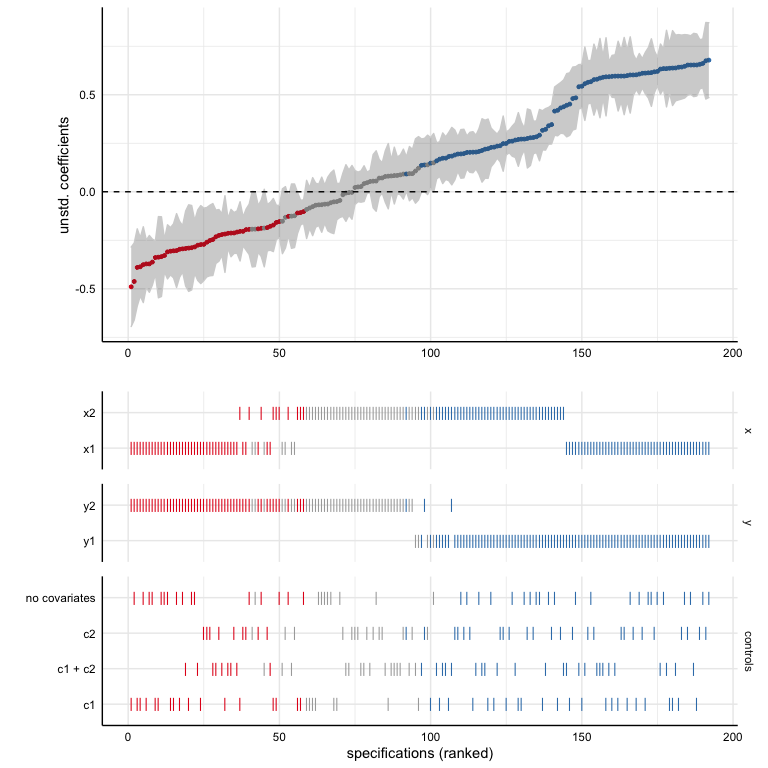### Include sample size histogram

By default, we do not know how many participants were included in each specification. If you removed missing values listwise beforhand, this may not be a big problem as all models are based on the same subsample. If you have missing values in your dataset and you did not impute them or delete them listwise, we should investigate how many participants were included in each specification. Using plot(x, type = "samplesizes"), we get a barplot that shows the sample size per specification.

p3 <- plot(results, type = "samplesizes")

plot_grid(p1, p2, p3,
ncol = 1,
align = "v",
axis = "rbl",
rel_heights = c(1.5, 2, 0.8))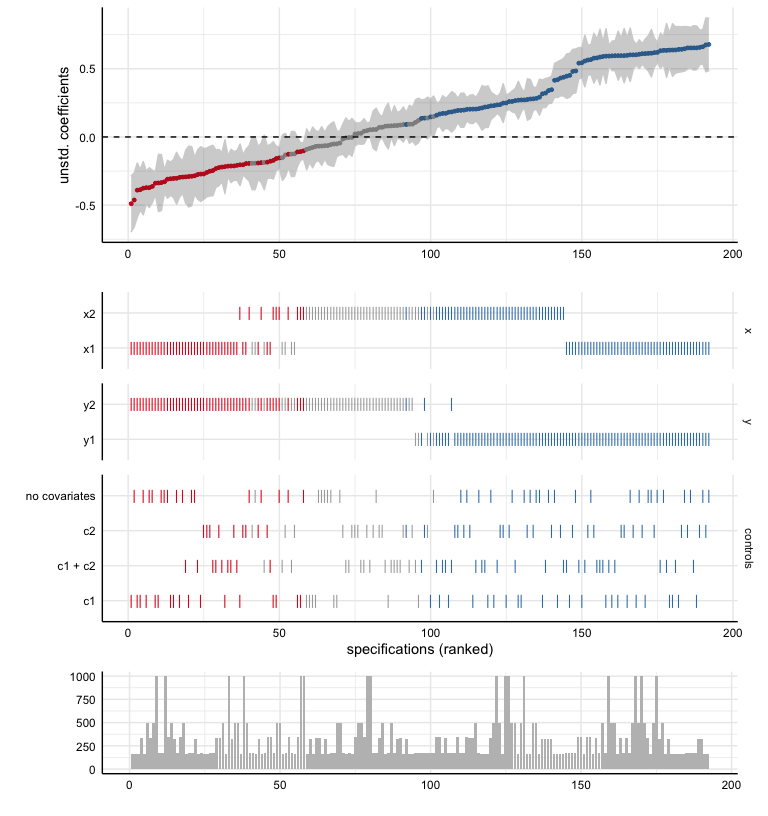## Alternative way to visualize specification results

Although the above created figure is by far the most used in studies using specification curve analysis, there are alternative ways to visualize the results. As the parameters resulting from the different specifications are in fact a distribution of parameters, a box-n-whisker plot (short “boxplot”) is a great alternative to investigate differences in results contingent on analytical chocies. We simply have to use the argument type = "boxplot" to create such plots.

# ALl choices
plot(results,
type = "boxplot")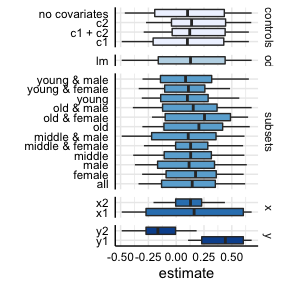# Specific choices and further adjustments
plot(results,
type = "boxplot",
choices = c("x", "y", "controls")) +
scale_fill_brewer(palette = 4)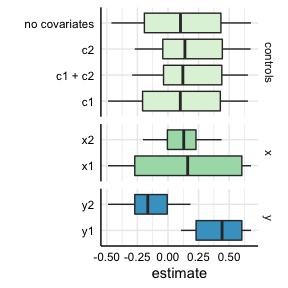plot(results,
type = "boxplot",
choices = c("group1", "group2")) +
scale_fill_manual(values = c("steelblue", "darkred"))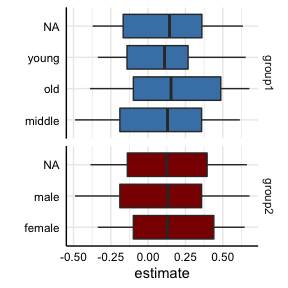Bear in mind, however, that next to these generic functions, we can always use the resulting data frame and plot our own figures.

results %>%
as_tibble %>%
ggplot(aes(x = group1, y = estimate, fill = group2)) +
geom_boxplot() +
scale_fill_brewer(palette = "Pastel1") +
theme_classic() +
labs(x = "age groups", fill = "gender")